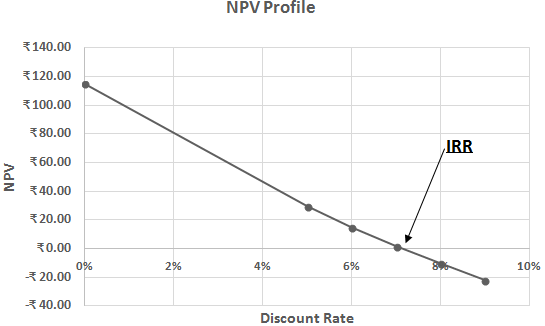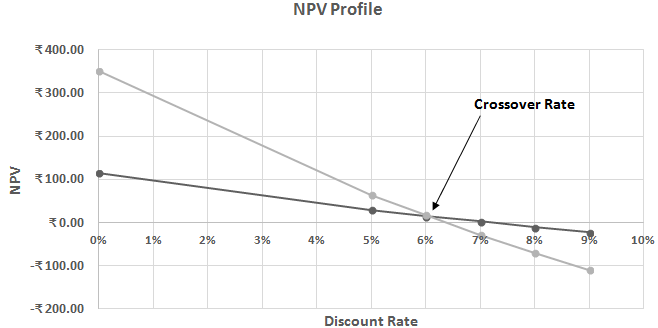# Net Present Value Profile (NPV Profile)

NPV Profile is the graphical representation of NPV at various discount rates. This is used to study the trend in variation of NPV with respect to the Discount Rate.
• NPV Profile is also used to study the crossover rate of various projects. Crossover Rate is the discount rate at which the net present values of two projects are equal. It is the point at which the NPV profile of one project intersects the NPV profile of the other project.
Illustration 1: ABC Ltd has invested Rs. 500 in a project for 5 years that generates cash flow over the period. Let us see how the NPV Profile looks for this case.

The various discount rates considered are 0%,5%,6%,7%,8%,9% and the project generates cash flow as below:
 Year Net Cash Flow (Rs.) 1 2 3 4 5 110 130 120 120 135• The point at which the line intersects the X-axis is the IRR for this cash flow.
• The point at which the line intersects the Y-axis is the NPV when the discount rate is 0%.
Illustration 2: XYZ Ltd has two investment proposals, the details of which are given below, let us observe its NPV profile. The discount rates are 0%,5%,6%,7%,8%,9%
 Initial Investment Year 1 Year 2 Year 3 Year 4 Year 5 Net Cash Flow Investment 1 500 110 130 120 120 135 Net Cash Flow Investment 2 2000 500 600 650 400 200• The point at which both lines intersect each other is the Crossover Rate.